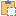#### Room Class

Namespace: Autodesk.Revit.DB.Architecture
Assembly: RevitAPI (in RevitAPI.dll) Version: 16.0.0.0 (16.0.0.0)

# Syntax

C#
``public class Room : SpatialElement``
Visual Basic
``````Public Class Room _
Inherits SpatialElement``````
Visual C++
``public ref class Room : public SpatialElement``

# Remarks

The room object can be queried for its boundary for use in space planning tools.

# ExamplesCopy C#
``````private void Getinfo_Room(Room room)
{
string message = "Room: ";

//get the name of the room
message += "\nRoom Name: " + room.Name;

//get the room position
LocationPoint location = room.Location as LocationPoint;
XYZ point = location.Point;
message += "\nRoom position: " + XYZToString(point);

//get the room number
message += "\nRoom number: " + room.Number;

IList<IList<Autodesk.Revit.DB.BoundarySegment>> segments = room.GetBoundarySegments(new SpatialElementBoundaryOptions());
if (null != segments)  //the room may not be bound
{
foreach (IList<Autodesk.Revit.DB.BoundarySegment> segmentList in segments)
{
message += "\nBoundarySegment of the room: ";
foreach (Autodesk.Revit.DB.BoundarySegment boundarySegment in segmentList)
{
// Get curve start point
XYZ start = boundarySegment.GetCurve().GetEndPoint(0);
message += "\nCurve start point: " + XYZToString(start);
// Get curve end point
XYZ end = boundarySegment.GetCurve().GetEndPoint(1);
message += " Curve end point: " + XYZToString(end);

// Show the boundary elements
message += "\nBoundary element id: " + boundarySegment.ElementId.IntegerValue;
}
}
}

}

// output the point's three coordinates
string XYZToString(XYZ point)
{
return "(" + point.X + ", " + point.Y + ", " + point.Z + ")";
}``````Copy VB.NET
``````Private Sub Getinfo_Room(room As Room)
Dim message As String = "Room: "

'get the name of the room
message += vbLf & "Room Name: " & Convert.ToString(room.Name)

'get the room position
Dim location As LocationPoint = TryCast(room.Location, LocationPoint)
Dim point As XYZ = location.Point
message += vbLf & "Room position: " & XYZToString(point)

'get the room number
message += vbLf & "Room number: " & Convert.ToString(room.Number)

Dim segments As IList(Of IList(Of Autodesk.Revit.DB.BoundarySegment)) = room.GetBoundarySegments(New SpatialElementBoundaryOptions())
If segments IsNot Nothing Then
'the room may not be bound
For Each segmentList As IList(Of Autodesk.Revit.DB.BoundarySegment) In segments
message += vbLf & "BoundarySegment of the room: "
For Each boundarySegment As Autodesk.Revit.DB.BoundarySegment In segmentList
' Get curve start point
Dim start As XYZ = boundarySegment.GetCurve().GetEndPoint(0)
message += vbLf & "Curve start point: " & XYZToString(start)
' Get curve end point
Dim [end] As XYZ = boundarySegment.GetCurve().GetEndPoint(1)
message += " Curve end point: " & XYZToString([end])

' Show the boundary elements
message += vbLf & "Boundary element id: " + boundarySegment.ElementId.IntegerValue
Next
Next
End If